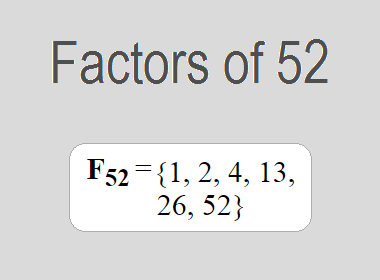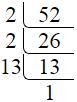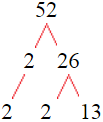# Factors of 52The factors of 52 are 1, 2, 4, 13, 26, and 52 i.e. F52 = {1, 2, 4, 13, 26, 52}. The factors of 52 are all the numbers that can divide 52 without leaving a remainder.

We can check if these numbers are factors of 52 by dividing 52 by each of them. If the result is a whole number, then the number is a factor of 52. Let's do this for each of the numbers listed above:

·        1 is a factor of 52 because 52 divided by 1 is 52.

·        2 is a factor of 52 because 52 divided by 2 is 26.

·        4 is a factor of 52 because 52 divided by 4 is 13.

·        13 is a factor of 52 because 52 divided by 13 is 4.

·        26 is a factor of 52 because 52 divided by 26 is 2.

·        52 is a factor of 52 because 52 divided by 52 is 1.

## How to Find Factors of 52?

1 and the number itself are the factors of every number. So, 1 and 52 are two factors of 52. To find the other factors of 52, we can start by dividing 52 by the numbers between 1 and 52. If we divide 52 by 2, we get a remainder of 0. Therefore, 2 is a factor of 52. If we divide 52 by 3, we get a remainder of 1. Therefore, 3 is not a factor of 52.

Next, we can check if 4 is a factor of 52. If we divide 52 by 4, we get a remainder of 0. Therefore, 4 is also a factor of 52. We can continue this process for all the possible factors of 52.

Through this process, we can find that the factors of 52 are 1, 2, 4, 13, 26, and 52. These are the only numbers that can divide 52 without leaving a remainder.

********************

********************

## Properties of the Factors of 52

The factors of 52 have some interesting properties. One of the properties is that the sum of the factors of 52 is equal to 98. We can see this by adding all the factors of 52 together:

1 + 2 + 4 + 13 + 26 + 52 = 98

Another property of the factors of 52 is that the prime factors of 52 are 2 and 13.

## Applications of the Factors of 52

The factors of 52 have several applications in mathematics. One of the applications is in finding the highest common factor (HCF) of two or more numbers. The HCF is the largest factor that two or more numbers have in common. For example, to find the HCF of 52 and 65, we need to find the factors of both numbers and identify the largest factor they have in common. The factors of 52 are 1, 2, 4, 13, 26, and 52. The factors of 65 are 1, 5, 13, and 65. The largest factor that they have in common is 13. Therefore, the HCF of 52 and 65 is 13.

Another application of the factors of 52 is in prime factorization. Prime factorization is the process of expressing a number as the product of its prime factors. The prime factors of 52 are 2 and 13, since these are the only prime numbers that can divide 52 without leaving a remainder. Therefore, we can express 52 as:

52 = 2 × 2 × 13

We can do prime factorization by division and factor tree method also. Here is the prime factorization of 52 by division method,52 = 2 × 2 × 13

Here is the prime factorization of 52 by the factor tree method,52 = 2 × 2 × 13

## Conclusion

The factors of 52 are the numbers that can divide 52 without leaving a remainder. The factors of 52 are 1, 2, 4, 13, 26, and 52. The factors of 52 have some interesting properties, such as having a sum of 98. The factors of 52 have several applications in mathematics, such as finding the highest common factor and prime factorization.